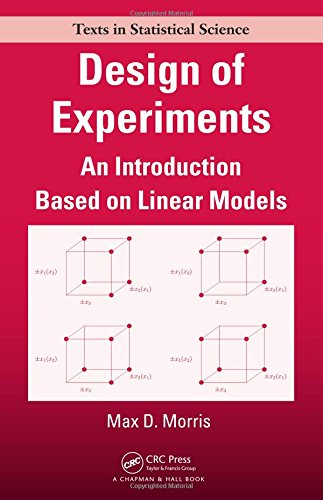# Design of Experiments: An Introduction Based on Linear Models

• Length: 376 pages
• Edition: 1
• Publisher:
• Publication Date: 2010-07-27
• ISBN-10: 1584889233
• ISBN-13: 9781584889236
5.0
Description

Offering deep insight into the connections between design choice and the resulting statistical analysis, Design of Experiments: An Introduction Based on Linear Models explores how experiments are designed using the language of linear statistical models. The book presents an organized framework for understanding the statistical aspects of experimental design as a whole within the structure provided by general linear models, rather than as a collection of seemingly unrelated solutions to unique problems.

The core material can be found in the first thirteen chapters. These chapters cover a review of linear statistical models, completely randomized designs, randomized complete blocks designs, Latin squares, analysis of data from orthogonally blocked designs, balanced incomplete block designs, random block effects, split-plot designs, and two-level factorial experiments. The remainder of the text discusses factorial group screening experiments, regression model design, and an introduction to optimal design. To emphasize the practical value of design, most chapters contain a short example of a real-world experiment. Details of the calculations performed using R, along with an overview of the R commands, are provided in an appendix.

This text enables students to fully appreciate the fundamental concepts and techniques of experimental design as well as the real-world value of design. It gives them a profound understanding of how design selection affects the information obtained in an experiment.